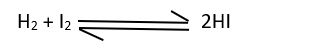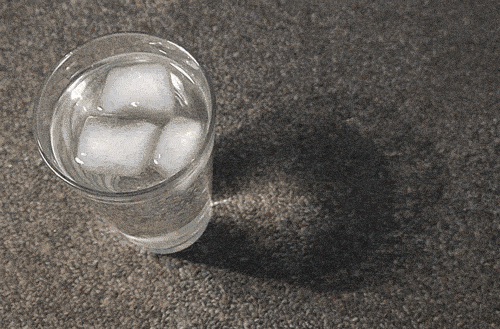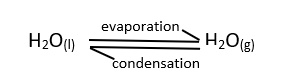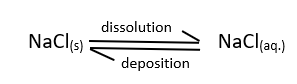# Chemical and Physical Equilibrium↪ A reversible reaction, whether physical or chemical, proceed to a limited extent and both reactants and products are available in the reaction mixture such that the concentrations of reactants and products remain constant after certain time.
↪ This process is called Equilibrium.
↪ This may be either chemical or physical equilibrium.
↪ The equilibrium in general is denoted as,Chemical Equilibrium↪ The stage of a reversible reaction at which the rate of forward reaction becomes equal to the rate of backward reaction.

⁕ Dynamic Nature of Chemical Equilibrium↪ The equilibrium is a dynamic balance between forward and backward reaction.
In dynamic equilibrium the forward and backward reaction proceed at equal speeds.↪ At equilibrium state, concentration of reactants and products do not change with time and reaction seems to be stopped but actually that is not so.
↪ The cause behind this is due to the equal rate of forward and backward reaction.
↪ Due to this chemical equilibrium is dynamic in nature rather than static.⁕ Characteristics of Chemical Equilibrium

↪ At equilibrium the concentration of the reactants and products become constant.
↪ At equilibrium the rate of forward reaction is equal to the rate of backward reaction and hence the equilibrium is dynamic in nature.
↪ A catalyst does not alter the rate of equilibrium.
↪ A chemical equilibrium is possible only in closed system.
↪ A chemical equilibrium is characterized by a constant called equilibrium constant (k).

Physical Equilibrium↪ The equilibrium achieved in physical process is called physical equilibrium.
↪ Some physical processes are also reversible process which are as follows:

⁕ Solid-Liquid Equilibrium

↪ Here exist equilibrium between solid and liquid phase.
↪ For example, equilibrium between ice and water at 0°C in an insulated thermos flask at one atmospheric pressure.↪ Rate of melting of ice ⇌ rate of freezing of water

⁕ Liquid-Gas Equilibrium

↪ The equilibrium between water and vapor in a closed vessel at normal temperature.⁕ Solid-Gas Equilibrium

↪ In case of volatile substance.
For example: Camphor
Camphor(s)Camphor(g)

⁕ Solute-Solution Equilibrium

↪ In this equilibrium the rate of dissolution is equal to the rate of deposition in a solution at particular temperature.
For example:
↪ Equilibrium between solid sodium chloride with sodium chloride solution.